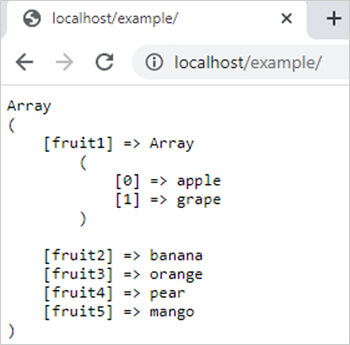array_merge_recursive() function in php || array merge recursive function

# array_merge_recursive() function in php

0 1387

array_merge_recursive() function in PHP is used to merge two or more arrays into a new array means it add the value of an array into the end of another array.

Note: the difference between array_merge and array_merge_recursive is that if two arrays have same keys then array_merge allocate the value of second array to that key but array_merge_recursive function assign a array to that key which contains values of both arrays assigned to that key.

Syntax:

array_merge_recursive(\$aar1,\$arr2,.....,\$arrN);

Here

\$arr1,\$arr2 and \$arrN are PHP arrays.

Example:

```<?php
\$arr1 = array("fruit1"=>"apple","fruit2"=>"banana","fruit3"=>"orange");
\$arr2 = array("fruit1"=>"grape","fruit4"=>"pear","fruit5"=>"mango");
\$result=array_merge_recursive(\$arr1,\$arr2);
echo "<pre>";
print_r(\$result);
?>```

Output:Share: